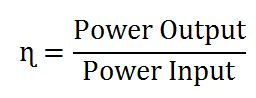## Block and Drilling Line Calculation

This article will focus on block and drilling line calculations as block efficiency, drilling power input/output, etc. Additionally, there are some examples which will help you understand how the formulas work.Block and drilling line efficiency formula is described below;Power Output (Po) = Fh x Vtb

Power Input (Pi) = Ff x Vf